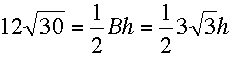SEARCH HOMEMath Central Quandaries & QueriesQuestion from linda, a student: IF AREA EQUALS 12radical 30 of the triangle find the height B= 3 radical 3Hi Again Linda,

If the height of the triangle is h and the base is B then the area isSolve for h using the fact that 30 = 3 × 10.

PennyMath Central is supported by the University of Regina and The Pacific Institute for the Mathematical Sciences.